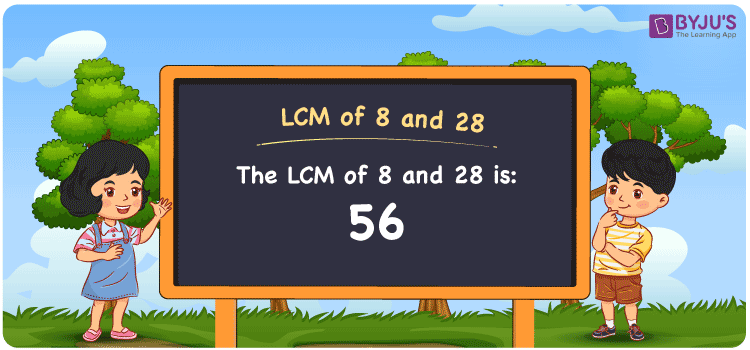# LCM of 8 and 28

LCM of 8 and 28 is 56. The smallest number divisible evenly among the multiples of the numbers 8 and 28 gives the LCM. The common multiples of the two numbers helps you find the least common multiple of 8 and 28. (8, 16, 24, 32, 40, 48, ….) and (28, 56, 84, 112, 140, 168,….) are the multiples of 8 and 28. The LCM of the given numbers using the prime factorisation method, division method and by listing out the multiples is explained briefly in this article.

Also read: Least common multiple

## What is LCM of 8 and 28?

The answer to this question is 56. The LCM of 8 and 28 using various methods is shown in this article for your reference. The LCM of two non-zero integers, 8 and 28, is the smallest positive integer 56 which is divisible by both 8 and 28 with no remainder.## How to Find LCM of 8 and 28?

LCM of 8 and 28 can be found using three methods:

• Prime Factorisation
• Division method
• Listing the multiples

### LCM of 8 and 28 Using Prime Factorisation Method

The prime factorisation of 8 and 28, respectively, is given by:

8 = 2 x 2 x 2 = 2³

28 = 2 x 2 x 7 = 2² x 7¹

LCM (8, 28) = 56

### LCM of 8 and 28 Using Division Method

We’ll divide the numbers (8, 28) by their prime factors to get the LCM of 8 and 28 using the division method (preferably common). The LCM of 8 and 28 is calculated by multiplying these divisors.

 2 8 28 2 4 14 2 2 7 7 1 7 x 1 1

No further division can be done.

Hence, LCM (8, 28) = 56

### LCM of 8 and 28 Using Listing the Multiples

To calculate the LCM of 8 and 28 by listing out the common multiples, list the multiples as shown below

 Multiples of 8 Multiples of 28 8 28 16 56 24 74 32 112 40 140 48 168 56 196 64 224

LCM (8, 28) = 56

## Video Lesson on Applications of LCM## LCM of 8 and 28 Solved Examples

Question: The LCM and GCD of two numbers are 56 and 4. If one number is 28, determine the other.

Solution:

Consider b as the other number

We know that

LCM x GCD = 28 x b

b = (LCM X GCD)/28

b = (56 x 4)/ 28

b = 8

Hence, the other number is 8.

## Frequently Asked Questions on LCM of 8 and 28

### Find the LCM of 8 and 28.

The LCM of 8 and 28 is 56.

The smallest multiple exactly divisible by 8 and 28 should be found.

Multiples of 8 = 8, 16, 24, 32, 40, 48, 56, …

Multiples of 28 = 28, 56, 84, 112, 140, ….

The smallest common multiple exactly divisible by 8 and 28 is 56.

### List the methods used to find the LCM of 8 and 28.

The methods used to find the LCM of 8 and 28 are the Prime Factorization Method, Division Method and Listing multiples.

### What is the GCF if the LCM of 8 and 28 is 56?

LCM x GCF = 8 x 28

Given

LCM of 8 and 28 = 56

56 x GCF = 224

GCF = 224/56 = 4

### What is the LCM of 8 and 28?

The LCM of 8 and 28 is 56.

### Find the LCM of 8 and 28 in 42, 32, 56, 100.

The LCM of 8 and 28 is the smallest common multiple which is divisible evenly by the given numbers. 56 satisfies this condition.